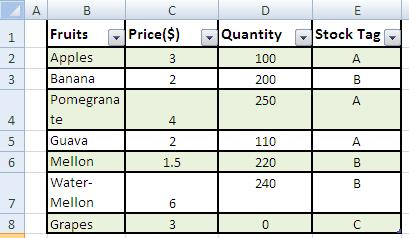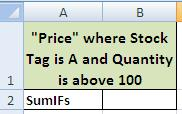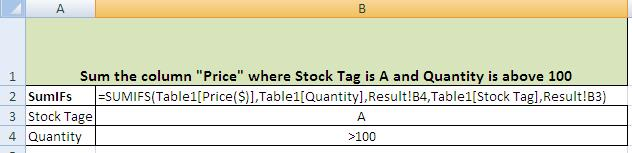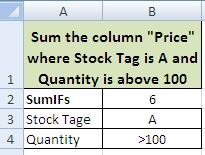# MS Excel using Sumifs easy way step by step

## Step by Step: How to Use SumIFS in excel 2003, 2007 & 2010 for Sum of values in a column on multiple, several or more than one condition or conditions.

MS Excel using Sumifs easy way: How to sum column data where certain conditions meet? Excel has SumIFs Function that sums up data cells where cells contain digit or numbers in the column on which this formula is applied. A user has to specify certain condition for this purpose. SUMIF is a great and useful formula for taking sum of values which meet certain criteria specified elsewhere.

### Step 1: Using Sumifs

(b) Formulate your tables: these may be on the same table or on a different worksheet or even different workbooks.
(c) For the sake of example we have created two Tables in c and e:
(d) Table from where data is to be retrieved:excel sumifs function

(e) Table where data is to be fetched/desired.Ms Excel Sumifs

### Step 2: MS Excel SUMIFS

Ensure spellings for search values are the same in both the tables.

### Step 3: MS Excel SUMIFS

Make sure there is no extra space.

### Step 4: Using MS Excel SUMIFS Functions: Review the stepos of forumulaMS Excel 2003 2007 2010 SumifsMS Excel Sumifs function

### Result:Getting results from SUMIFS FormulaSumifs function Excel